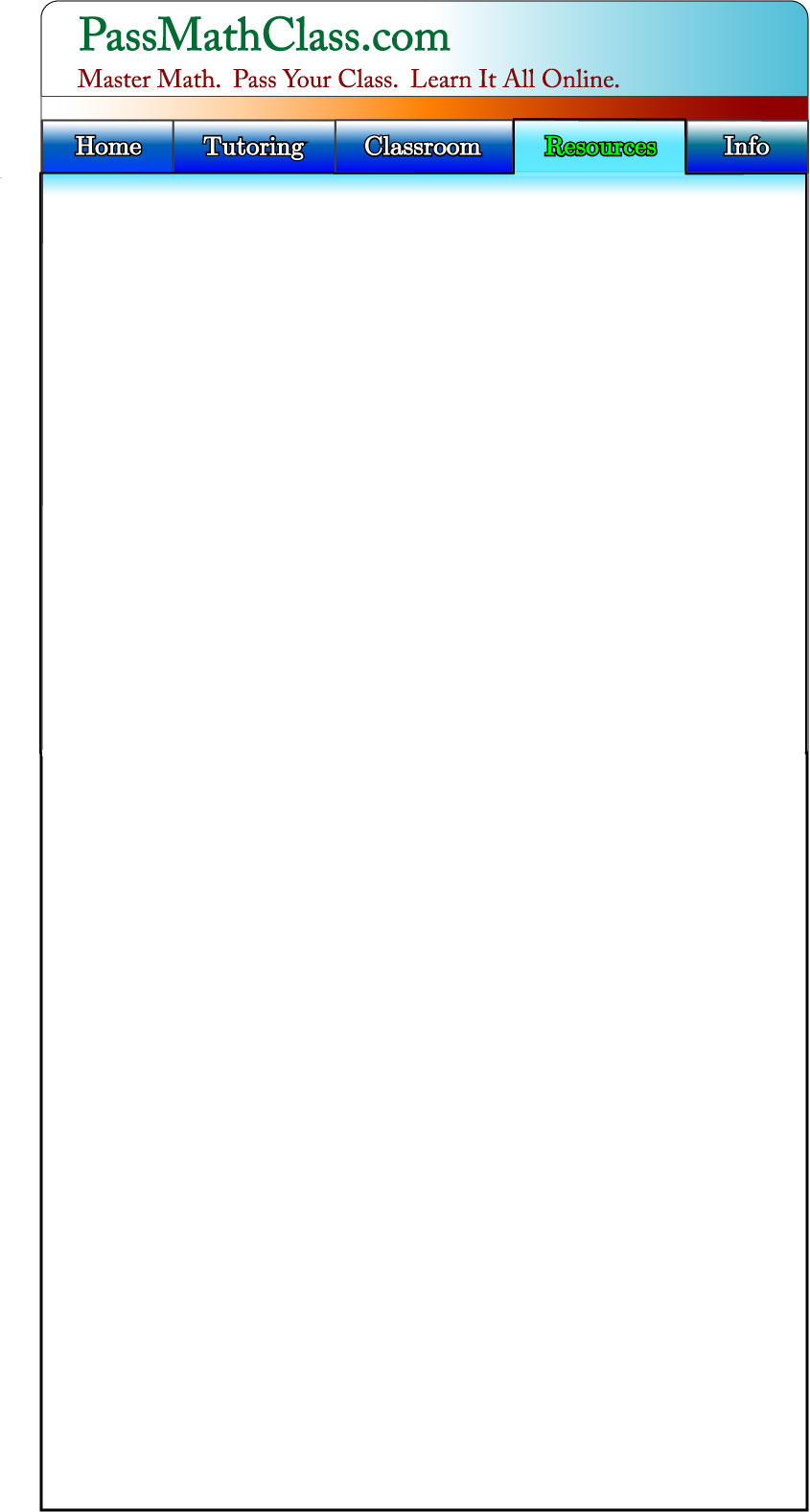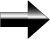The Foundation For Algebra

This section will familiarize you with the basic terms and concepts that algebra is built upon.  As you are completing the other lessons, make sure to come back and review this section if there is a concept that you are unfamiliar with.

Algebra is the basic way to manipulate equations.  It is the way we solve math problems.  It is a way of thinking, a tool to solve problems.

An Introduction To Numbers

In mathematics, we classify numbers into different groups in order to help us understand them:

Whole Numbers:  Whole numbers are numbers like 1, 2, and 3. They are “Whole” in the sense that they do not contain any fractions of numbers. The Whole Numbers are : 1, 2, 3, 4, and keep going forever.

Integers:  Integers include all the Whole Numbers, but also negatives.  -4, -3, … 0, 1, 2, ect.

Rational Numbers:  Rational Numbers are fractions.  Basically the ratio of two integers.  ½ is a rational number, as is 3 2/3 (11/3).

Irrational Numbers:  An Irrational number is a number with decimals that has no pattern.  Pi (π) is a classic example of an irrational number.  It goes on forever and has no pattern to it (3,14159 …) An Irrational Number can not be expressed as a ratio.  Note the difference between a number like 12/99 (0.1212121212121212), which goes on forever but has a pattern, and a number like PI that also goes on forever, but has no pattern.  12/99 is a Rational Number because it can be expressed as a fraction, a ratio of 2 integers.

Real Numbers:  The Real Numbers include all of the above types of numbers.  They include all the numbers you use in your everyday life.

Imaginary Numbers: Imaginary numbers are a very special type of number, and are defined based on the concept that i2 = -1.  As we will see, they are different than the real numbers.  We will deal with Imaginary numbers in a separate section.